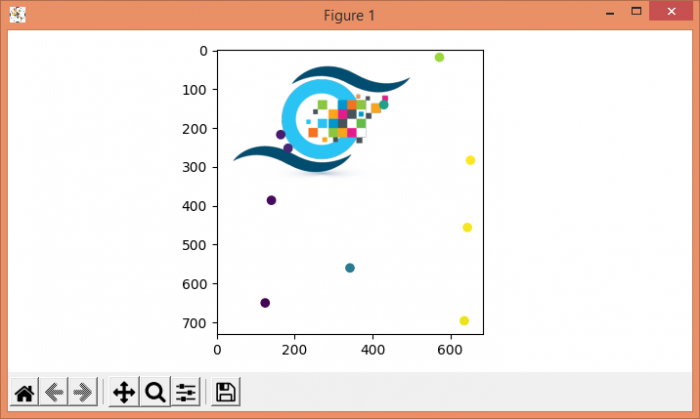# How do you directly overlay a scatter plot on top of a jpg image in Matplotlib?

To directly overlay a scatter plot on top of a jpg image, we can take the following steps −

• Load an image "bird.jpg", using imread() method, Read an image from a file into an array.

• Now display data as an image.

• To plot scatter points on the image make lists for x_points and y_points.

• Generate random numbers for x and y and append in lists.

• Using scatter method, plot x and y points.

• To display the figure, use show() method.

## Example

import numpy as np
from matplotlib import pyplot as plt
plt.rcParams["figure.figsize"] = [7.00, 3.50]
plt.rcParams["figure.autolayout"] = True
im = plt.imshow(data)
x_points = []
y_points = []
for i in range(10):
x_points.append(np.random.randint(0, 700))
y_points.append(np.random.randint(0, 700))
plt.scatter(x_points, y_points, c=x_points)
plt.show()

## Output